Multidimensional Integral over Irregular Domains

We will attempt to model the energy of ion just above one half of a triangular capacitor.  Suppose there is a uniformly charged surface  (σ≡charge/area=1) occupying an equilaterial triangle in the z=0 plane: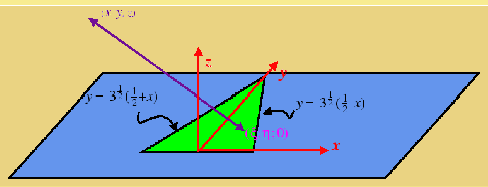what is the energy (voltage) of a unit positive charge located at (x,y,z)

The electrical potential goes like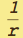, therefore the potential of a unit charge located at (x,y,z) from a small surface patch at (ξ,η,0) is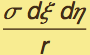=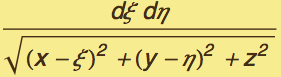Therefore it remains to integrate this function over the domain η∈(0,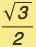) and ξ∈ (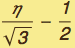) , (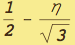))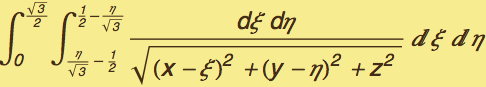First we investigate how Mathematica deals with multiple integrals: It integrates over the last iterator first: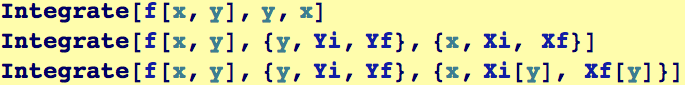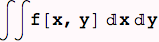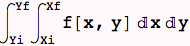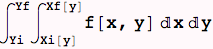For example,consider the difference in the following two cases:
First, we integrate over x and y using the two iterators in Integrate with the order {y,0,1}, {x,0,y}. Tnen explicitely using two separate steps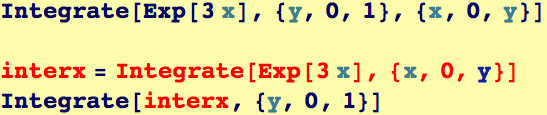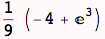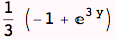Compared to
integrate over x and y using the two iterators in Integrate with the order {x,0,y},{y,0,1}. Tnen explicitely using two separate steps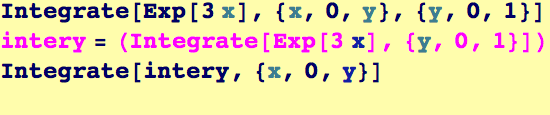Created by Wolfram Mathematica 6.0  (24 September 2007)